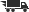Close

# Oscillation Theory for Difference and Functional Differential Equations

## by Ravi P. Agarwal

• ISBN: 9780792362890
• ISBN10: 0792362896

# Oscillation Theory for Difference and Functional Differential Equations

## by Ravi P. Agarwal

• List Price: \$174.00
• Binding: Hardcover
• Publish date: 05/01/2000
• ISBN: 9780792362890
• ISBN10: 0792362896
used \$119.33
You save: 31%
new \$123.96
You save: 29%
FREE economy shipping!
Description: Preface. 1. Oscillation of Difference Equations. 1.1. Introduction. 1.2. Oscillation of Scalar Difference Equations. 1.3. Oscillation of Orthogonal Polynomials. 1.4. Oscillation of Functions Recurrence Equations. 1.5. Oscillation in Ordered Sets. 1.6. Oscillation in Linear Spaces. 1.7. Oscillation in Archimedean Spaces. 1.8. Oscillation of Partial Recurrence Equations. 1.9. Oscillation of System of Equations. 1.10. Oscillation Between Sets. 1.11. Oscillation of Continuous-Discrete Recurrence Equations. 1.12. Second Order Quasilinear Difference Equations. 1.13. Oscillation of Even Order Difference Equations. 1.14. Oscillation of Odd Order Difference Equations. 1.15. Oscillation of Neutral Difference Equations. 1.16. Oscillation of Mixed Difference Equations. 1.17. Difference Equations Involving Quasi-differences. 1.18. Difference Equations with Distributed Deviating Arguments. 1.19. Oscillation of Systems of Higher Order Difference Equations. 1.20. Partial Difference Equations with Continuous Variables. 2. Oscillation of Functional Differential Equations. 2.1. Introduction. 2.2. Definitions, Notations and Preliminaries. 2.3. Ordinary Difference Equations. 2.4. Functional Difference Equations. 2.5. Comparison of Equations of the Same Form. 2.6. Comparison of Equations with Others of Lower Order. 2.7. Further Comparison Results. 2.8. Equations with Middle Term of Order (n - 1). 2.9. Forced Differential Equations. 2.10.Forced Equations with Middle Term of Order (n - 1). 2.11. Superlinear Forced Equations. 2.12. Sublinear Forced Equations. 2.13. Perturbed Functional Equations. 2.14. Comparison of Neutral Equations with Nonneutral Equations. 2.15 Comparison of Neutral Equations with Equations of the Same Form. 2.16. Neutral Differential Equations of Mixed Type. 2.17. Functional Differential Equations Involving Quasi-derivatives. 2.18. Neutral and Damped Functional Differential Equations Involving Quasi-derivatives. 2.19. Forced Functional Differential Equations Involving Quasi-derivatives. 2.20. Systems of Higher Order Functional Differential Equations. References. Subject Index.
Expand description
 Seller: HPB-Ohio ★★★★★ ★★★★★ Location: Columbus, OH Condition: GoodItem may show signs of shelf wear. Pages may include limited notes and[...] Item may show signs of shelf wear. Pages may include limited notes and highlighting. May include supplemental or companion materials if applicable. Access codes may or may not work. Connecting readers since 1972. Customer service is our top priority. Price: \$119.33 Comments: Item may show signs of shelf wear. Pages may include limited notes and[...] Item may show signs of shelf wear. Pages may include limited notes and highlighting. May include supplemental or companion materials if applicable. Access codes may or may not work. Connecting readers since 1972. Customer service is our top priority.